# Types of data

Here you will learn about types of data, including, qualitative data, quantitative data, discrete data, and continuous data.

Students first learn to work with data in first grade and expand their knowledge and use of data as they progress through the grades.

## What are types of data?

Types of data are collections of information to be sorted and used to understand things.

Data is used in many different ways that are useful.

We need to understand the different types of data that can be collected and the different statistical ways it can be analyzed or interpreted.

Quantitative Data:Qualitative Data:

This is numerical information that can be counted
or measured. You can quantify or give a numerical
value to the information.

Some of the ways you can collect quantitative
data is through experiments, polls, interviews,
surveys, or questionnaires.

This is non-numerical information that describes

Some of the ways you can collect qualitative
data are through questionnaires, surveys,
interviews, or observations.

Types of Quantitative Data:Types of Qualitative Data:

● Discrete data – data that is represented by a
specific set of numbers that are typically whole
numbers.

Examples of discrete data:
○ Number of students in a specific grade
○ Number of employees in a department
○ Number of products in a store

● Continuous data – data that can be measured.

Examples of Continuous data:
○ Height or weight of people in of a
particular age
○ Daily temperature in a city
○ Time needed to complete a specific task

● Categorical data – data that can be grouped
into categories instead of being measured
numerically.

Examples of discrete data:
○ Favorite color
○ Brands
○ Hair color
○ Eye color

Step-by-step guide: Qualitative data

Step-by-step guide: Quantitative data

Step-by-step guide: Discrete data

### What are types of data?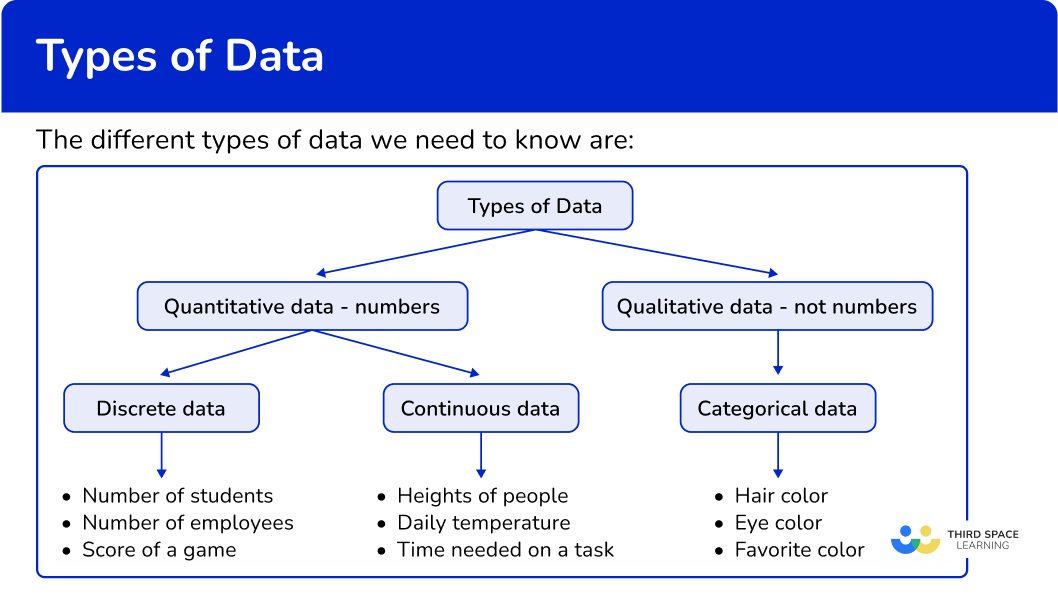## Common Core State Standards

How does this apply to 6th grade math?

• Grade 6 – Statistics and Probability (6.SP.A.1)
Recognize a statistical question as one that anticipates variability in the data related to the question and accounts for it in the answers. For example, “How old am I?” is not a statistical question, but “How old are the students in my school?” is a statistical question because one anticipates variability in students’ ages.

• Grade 6 – Statistics and Probability (6.SP.B.5)
Summarize numerical data sets in relation to their context, such as by: a. Reporting the number of observations.

## How to recognize types of data

There are several ways to classify data. For more specific step-by-step guides, check out the pages linked in the “What are types of data?” section above or read through the examples below.

In order to recognize types of data:

1. Recall the definition.
2. State and explain the type of data.

## Types of data examples

### Example 1: recognizing types of data

Jill wants to survey her classmates on the types of pets they have. Is this data qualitative or quantitative?

1. Recall the definition.

Qualitative data is non-numerical data.

Quantitative data is numerical data.

2State and explain the type of data.

Collecting data on types of pets is not numerical data. It’s data that represents the types of animals her classmates have as pets, so it is qualitative data.

### Example 2: recognizing types of data from a frequency graph

Mrs. Summerer’s class is going to do a data project on skittles. The students will gather data to figure out which color skittle is most represented in the bags of skittles that are sold. The bar graph by one student is shown below. Does the bar graph represent categorical data or continuous data?

Recall the definition.

State and explain the type of data.

### Example 3: recognizing types of data

Charlee does a survey in her class on favorite soda drinks. What type of data is Charlee collecting?

Recall the definition.

State and explain the type of data.

### Example 4: recognizing types of data from a frequency graph

The dot plot below represents the time it takes 40 students to eat breakfast. Use two words that describe the data in this dot plot.

Recall the definition.

State and explain the type of data.

### Example 5: recognizing types of data from a frequency graph

Mr. Shockely’s statistics class was conducting an experiment on rolling a die. The bar chart represents the data from a student rolling a die 40 times. What are two ways to describe the data?

Recall the definition.

State and explain the type of data.

### Example 6: recognizing types of data from a histogram

The histogram represents the heights of trees on a farm. Does the data in the histogram represent quantitative discrete data or quantitative continuous data?

Recall the definition.

State and explain the type of data.

### Teaching tips for types of data

• Incorporate project based data science learning activities where students have opportunity to collect their own data as well as do data analysis/statistical analysis on the collected data.

• Show students how to create data visualizations using Excel or Google Sheets.

### Easy mistakes to make

• Mixing up data types
It is very easy for students to mix up the different types of data. Using diagrams such as flow charts helps students recall the different types of data.

• Confusing continuous and discrete data
Understanding the difference between continuous and discrete data can be confusing for students. For example, shoe size is considered discrete data, not continuous data.

Even though a person’s shoe size is based on the length of their foot, the shoe size for a person can only be a certain set of values, such as an 8 or 8.5 in US sizing. Therefore, shoe size is an exact value, which makes it discrete data.

### Practice types of data questions

1. Farah was doing research on rainfall. The data she found was the amount of rainfall in millimeters that fell in Australia over the last 30 years.

Which option best describes the type of data she found?

QualitativeDiscreteContinuousCategoricalThe data she collected is numerical and based on measurement in millimeters. So, the data is continuous since continuous data is data that is measured.

2. The dot plot below represents Mrs. Smith’s students favorite type of lunch. Which of the following best describes the data?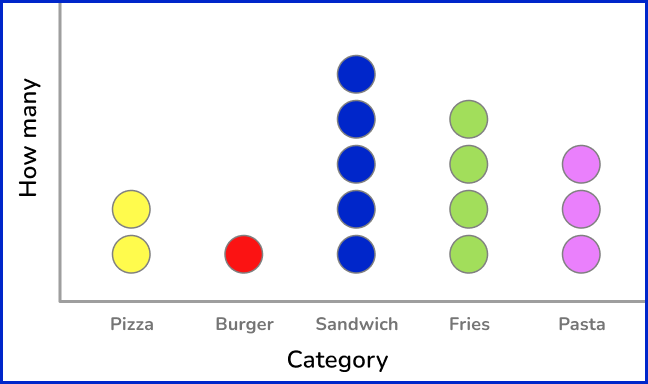Quantitative discrete dataQualitative discrete dataQuantitative continuous dataQualitative categorical dataThe data in the dot plot is not numerical and is grouped into categories of the types of food the students prefer for lunch. So, the data is qualitative categorical data.

3. The pie chart below shows the types of pets a group of 6th grade students have. Which of the following best describes the data?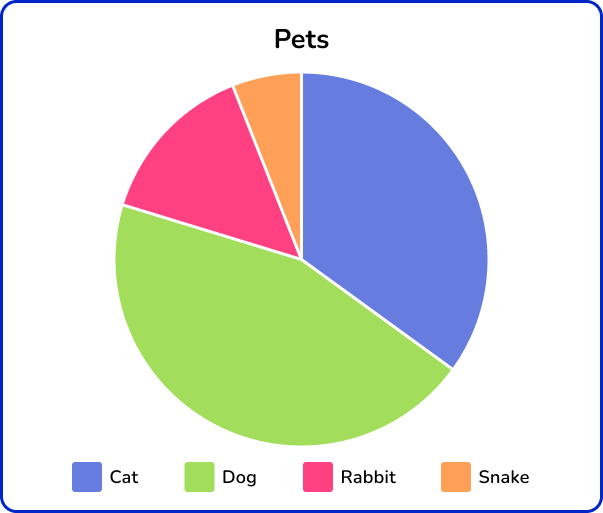QualitativeDiscreteContinuousQuantitativeThe pie chart represents non-numerical data based on categories of types of pets. So, the data is qualitative.

4. Simon did an experiment measuring the length of a spring based on the force applied to stretch it.

Which option best describes the type of data he collected?

Qualitative and categoricalQuantitative and categoricalQuantitative and continuousQuantitative and discreteThe data he collected is numerical and a measurement so it’s quantitative and continuous.

5. What type of data does the graph below represent?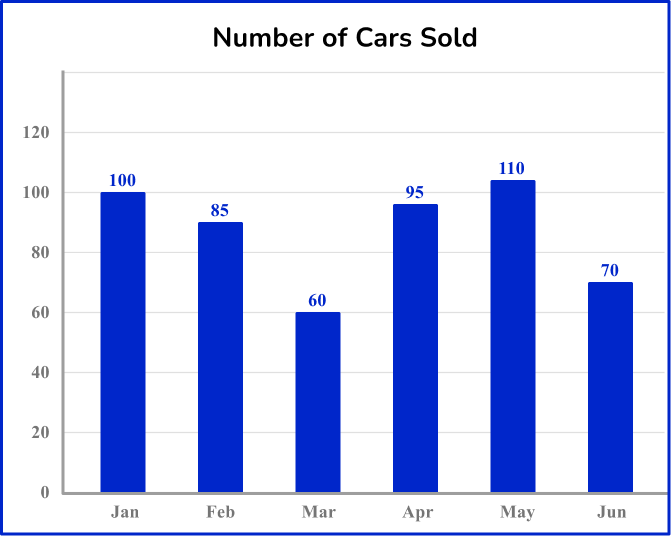Quantitative and discreteQuantitative and continuousQuantitative and categoricalQualitative and categoricalThe data in the bar chart represent numerical data. The data is also represented as exact values and is not a measurement. So, the data is quantitative and discrete.

6. Which option best describes the data shown on the frequency table.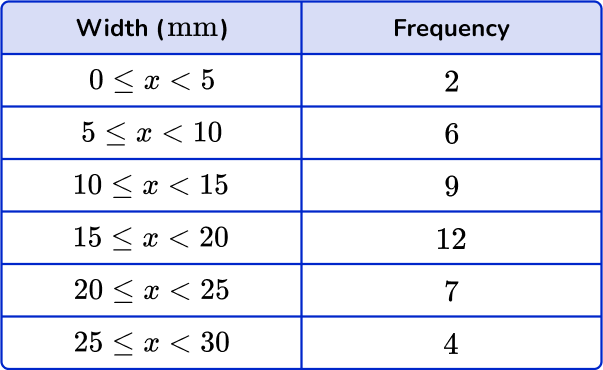QualitativeQuantitative and continuousQuantitative and discreteCategoricalThe data are numerical values and measurements.

## Types of data FAQs

What is primary data?

Primary data is data collected by the person conducting the actual research.

What is secondary data?

Secondary data is collected by another person who is not conducting the actual research.

What is nominal data?

Nominal data is a type of qualitative data. It cannot be ordered or measured. Examples of nominal data are zip code, blood type, and political party.

What is ordinal data?

Ordinal data is a type of qualitative data. It groups data into ordered categorical variables. Examples of ordinal data are socioeconomic status such as (low income, middle income, high income) or educational level (high school graduate, bachelor’s degree, master’s degree, doctorate).

What do data scientists do?

Data scientists need to know how to use programming languages; they do data management; they do exploratory data analysis; and use decision-making based on their data analysis.

What is descriptive statistics?

You will learn abaut descriptive statistics in high school. Descriptive statistics focuses on describing the visual characteristics of a particular data set.

What is big data?

Big data is larger, more complex data. Artificial intelligence and machine learning is used to do big data analytics.

Is statistics and data analysis a good field to study?

Statistical analysis/data analysis permeates many different career paths such as market research and customer satisfaction.

What is interval data?

Interval data is also known as an integer which means each data point is placed at equal distance from one another.

What is ratio data?

You will learn about ratio data in a statistics class. It is a type of quantitative data.

What is standard deviation?

You will learn about standard deviation in high school. It is the amount of variation of a data set.

## Still stuck?

At Third Space Learning, we specialize in helping teachers and school leaders to provide personalized math support for more of their students through high-quality, online one-on-one math tutoring delivered by subject experts.

Each week, our tutors support thousands of students who are at risk of not meeting their grade-level expectations, and help accelerate their progress and boost their confidence.